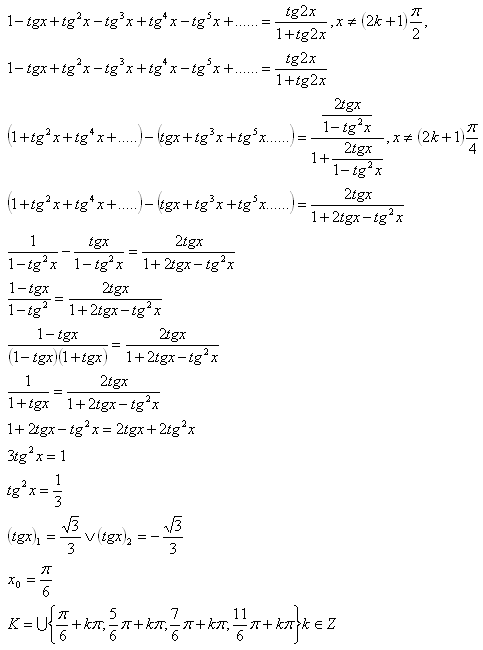sk|cz|

# Geometric Progression

1.Characterize a geometric progression:

Solution:
A progression (an)n=1 is told to be geometric if and only if exists such q є R real number; q ≠ 1, that for each n є N stands an+1 = an.q. Number q is called a geometric progression ratio.

Properties:
a) an = a1.qn-1
b) ar = as.qr-s
c)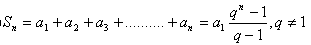d) Stable incrementation: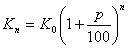e) Stable decrementation: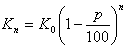f) Sum of an infinite geometric progression: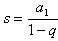q < 1

2.Determine first 6 members of a geometric progression if stands a3 = 8 and a7 = 128.

Solution: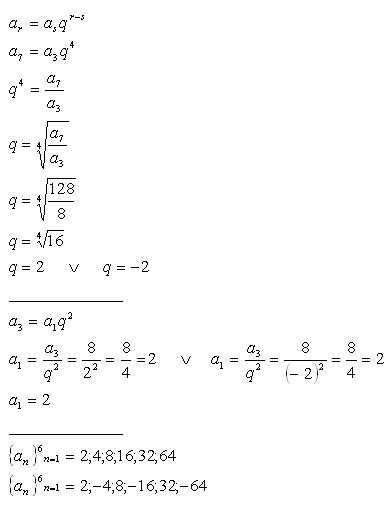3.If a number is added to 2, 16 and 58, it results in first 3 geometric progression members. Find out the number and enumerate first 6 members of the progression.

Solution: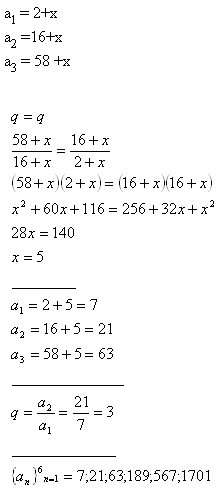4.Insert 4 numbers between the roots of the equation x2 -66x +128 = 0 so that they would make a geometric progression.

Solution: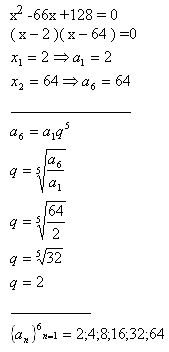5.Enumerate first 6 progression members of a geometric progression that fits following conditions: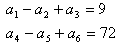Solution: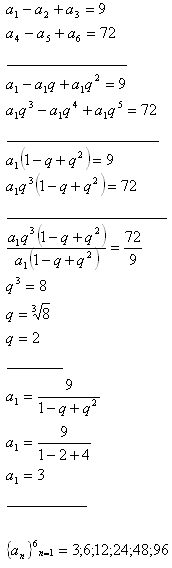6.The surface area of a cuboid equals S = 78 cm2. The sides of the cuboid make a geometric progression. Sum of the lengths of the sides intersecting in one of the edges is 13 cm. Determine the volume of the cuboid.

Solution: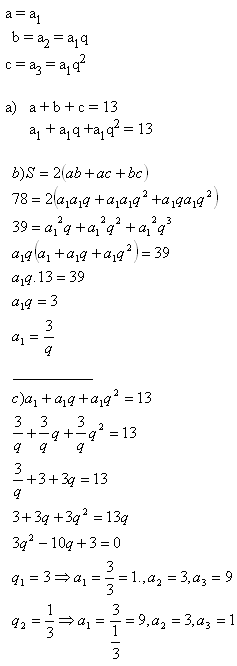The sides lengths are a = 1cm, b = 3cm, c = 9cm.
The volume equals V = a.b.c
V = 1.3.9 cm3
V = 27 cm3

7.An equestrian wants to buy a horse for \$10,000. He made a deal with the salesman to pay for the nails in the horseshoes. He paid 1 cent for the first nail, 2 cents for the second nail, 4 cents for the third nail and so on. Each horseshoe is fastened by 5 nails. Did he make a good deal?

Riešenie: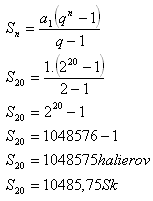The equestrian overbid the horse for \$485,75.

8.A workman agreed to work under following conditions: His salary for the first day of work will be \$1, for the second day of work \$2, for the third day of work \$4, and so on. How long does he have to work to earn \$4095?

Solution: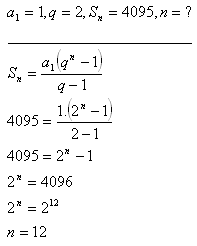The workman needs to work for 12 days.

9.What should be the bank interest to raise the deposit of \$10000 to \$25 000 in 5 years?

Solution: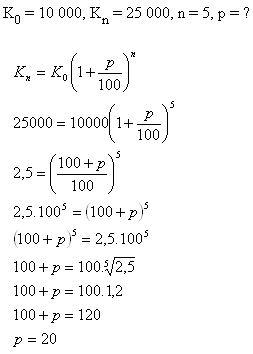The interest should be 20%.

10.What is the sum of following infinite geometric progression?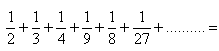Solution: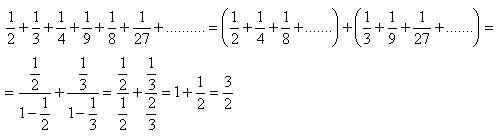The sum of the progression members is 3/2.

11. Solve in real numbers: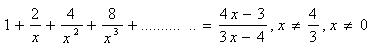Solution: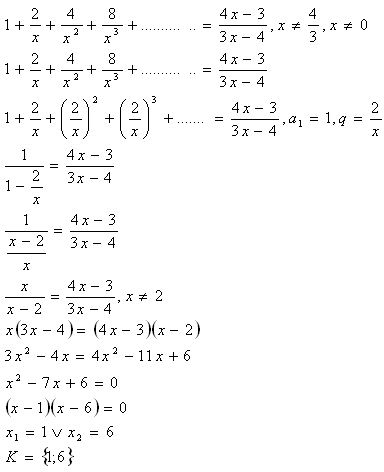12. Solve in real numbers: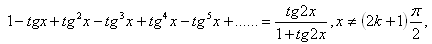Solution: